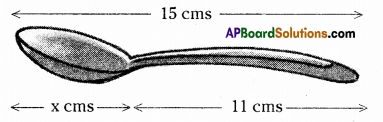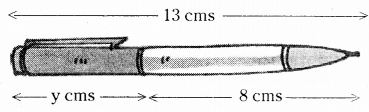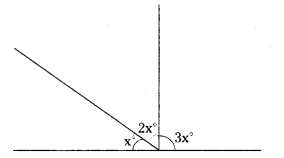# AP Board 7th Class Maths Solutions Chapter 3 Simple Equations Ex 3

AP State Syllabus AP Board 7th Class Maths Solutions Chapter 3 Simple Equations Ex 3 Textbook Questions and Answers.

## AP State Syllabus 7th Class Maths Solutions 3rd Lesson Simple Equations Exercise 3Question 1.
Write the information given in the picture in the form of an equation. Also, find ‘x’ in the following figure.Solution:
From the figure x + 11 = 15
∴ x = 15 – 11 (transposing +11)
∴ x = 4cm

Question 2.
Write the information given in the picture in the form of an equation. Also, find ‘y in the following figure.Solution:
From the figure y + 8 = 13
y = 13 – 8 (transposing 8)
∴ y = 5cmQuestion 3.
If we add 7 to twice a number, we get 49. Find the number.
Solution:
Let the number be x
Then twice the number = 2x
By problem, 2x + 7 = 49
2x = 49 – 7 (transposing + 7)
2x = 42
x = $$\frac { 42 }{ 2 }$$ (transposing x 2)
x = 21

Question 4.
If we subtract 22 from three times a number, we get 68. Find the number.
Solution:
Let the number be x
Then three times the number = 3x
On subtracting 22 ⇒ 3x – 22
By problem, 3x – 22 = 68
3x = 68 + 22 (transposing -22)
3x = 90
x = $$\frac { 90 }{ 3 }$$(transposingx3)
x = 30
∴ The required number 30

Question 5.
Find a number which when multiplied by 7 and then reduced by 3 is equal to 53.
Solution:
Let the number be x
Multiplied by 7 ⇒ 7x
Then reduced by 3 ⇒ 7x – 3
By problem, 7x – 3 = 53
7x = 53 + 3 (transposing – 3)
7x = 56
x = $$\frac { 56 }{ 7 }$$ (transposing x 7)
x = 8 .
∴ The required number = 8Question 6.
Sum of two numbers is 95. 1f one exceeds the other by 3, find the numbers.
Solution:
Let one number be = x.
Then the other number x – 3
Sumofthenumbers x + x – 3 = 2x – 3
By problem, 2x – 3 = 95
2x = 95 + 3 (transposing – 3)
2x = 98
x = $$\frac { 98 }{ 2 }$$ (transposing x 2)
x = 49
∴if one number x 49 then the other number x – 3 = 49 – 3 = 46Question 7.
Sum of three consecutive integers is 24. Find the integers.
Solution:
Let the three integers be = x, x + 1, x 2
Sumoftheintegers = x + x + 1 + x + 2 = 3x + 3
By problem, 3x + 3 = 24’
3x = 24 – 3 (Transposing + 3)
3x = 21
x = $$\frac { 21 }{ 3 }$$ (transposing x 3)
x = 7
∴ The integers x = 7
x + 1 = 7 + 1 = 8
x + 2 = 7 + 2 = 9

Question 8.
Find the length and breadth of the rectangle given below if its perimeter is 72m.Solution:
Length of the rectangle = 5x + 4
Breadth of the rectangLe = x – 4
Perimeter of the rectangle = 2 x (length + breadth)
= 2x[(5x + 4)+(x – 4)]
= 2[5x + 4 + x – 4]
= 2(6x)
= 12x
Byproblem, 12x = 72
x = $$\frac { 72 }{ 12 }$$ (transposing x 12)
x = 6
∴ Length of the rectangle = 5x + 4 = 5 x 6.4 = 34cm
Breadth of the rectangÌe = x – 4 = 6 – 4 = 2cmQuestion 9.
Length of a rectangle exceeds its breadth by 4 m. 1f the perimeter of the rectangle is 84 m, find its length and breadth.
Solution:
Then its length = x + 4
Perimeter of the rectangle = 2 x (length + breadth)
=2[x + 4 + x]
= 2 (2x + 4)
= 4x + 8
By problem, 4x + 8 = 84
4x = 84 – 8 (transposing + 8)
4x = 76
x = $$\frac{76}{4}$$ (transposing x 4)
x = 19
∴ Length of the rectangle =x+4= 19+4 = 23m
Breadth of the rectangle = 19m

Question 10.
After 15 years, Hema’s age will become four times that of her present age. Find her present age.
Solution:
Let the preser age of Hema be x years
After 15 years Hema Age = 4x
By problem, x + 15 = 4x
x + 15 – 4x = 4x – 4x (Subtracting 4x from both sides)
– 3x + 15 = 0
– 3x = – 15
x = $$\frac{-15}{-3}$$ transposing x ( – 3)]
x = 5
∴ Her present age is 5 years.
∴ Her present age is 5 years.Question 11.
A sum of ₹.3000 is to be given in the form of 63 prizes. Ifthe prize money is either ₹. 100 or.25. Find the number of prizes of each type.
Solution:
Let the number of ₹ 100 prizes be x
Then the number of ₹ 25 prizes be = 63 – x
Value of the prizes = 100x + (63 – x) x 25
= 100x+ 1575 – 25x
= 75x + 1575
By problem, 75x + 1575 = 3000
75x = 3000 – 1575
75x = 1425
x = $$\frac { 1425 }{ 75 }$$
x = 19
∴ ₹ 100 prizes = 19
₹25 prizes= 63 – x =63 – 19 = 44

Question 12.
A number is divided into two parts such that one part is 10 more than the other. Ifthe two parts are in the ratio 5:3, find the number and the two parts.
Solution:
Let one part be x
Then the other part = x + 10
Ratio of these two parts = x + 10 : x
Byproblem, x + 10 : x=5:3
∴ $$\frac{x+10}{x}=\frac{5}{3}$$
3(x+ 10)=5x
3x + 30 = 5x
30 = 5x – 3x
2x = 30
x = $$\frac{30}{2}$$
x = 15
If one part is 15 then the other part must be x + 10 = 15 + 10 = 25
∴ The number is 15 + 25 40Question 13.
Suhana said, “multiplying my number by 5 and adding 8 to it gives the same answer as subtracting my number from 20”. Find Suhana’s numbers.
Solution:
Let Suhana’s number be x
Muhtplying by S and adding 8 to that number 5x + 8
Subtracting that number from 20 = 20 – x
By problem above two are equal.
i.e. 5x + 8 = 20 – x
5x + x = 20 – 8
6x = 12
x = $$\frac{12}{6}$$ = 2
x = 2
∴ Suhanas number is 2

Question 14.
The teacher tells the class that the highest marks obtained by a student in her class is twice the lowest marks plus 7. The highest mark is 87. What is the lowest mark?
Solution:
Let the Lowest mark of the class = x
Twice the least mark = 2x
On adding 7 = 2x + 7
By problem; 2x + 7 = 87
2x = 87 – 7
2x = 80
x = $$\frac{80}{2}$$ = 40
x = 40
∴ The lowest mark = 40Question 15.
In adjacent figure find the magnitude of each of the three angles formed?
(Hint: Sum of all angles at a point on a line is 180°)
Solution:
(Hint : Sum of all angles at a point on a line is 180°)We know sum of angles at a point = 180°
∴ x° + 2x° + 3x° = 180
6x° = 180°
x = $$\frac{180}{6}$$ = 30
∴ The angles are x = 30°
2x = 2 x 30 = 60°
3x° = 3 x 30° = 90°

Question 16.
Solve the following riddle:
I am a number
Tell my identity
Take me two times over
2x = $$\frac{60}{2}$$